## GOING IN CIRCLES [DAY 5]

### EXPLORING PI: CIRCUMFERENCE & AREA OF CIRCLES

Leverage contextual problems to investigate and emerge Pi to help conceptualize formulas for both the circumference and area of a circle.

## Intentionality & Unit Overview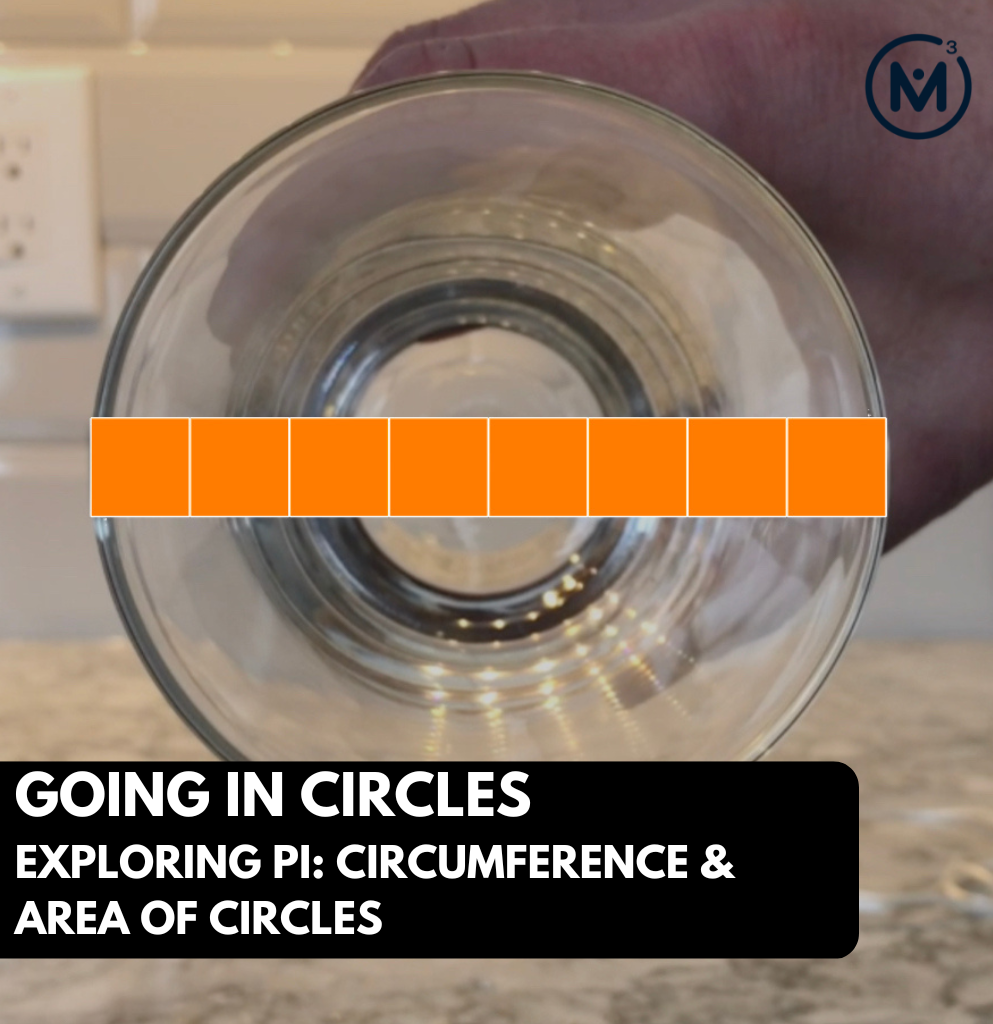### Access each lesson from this unit using the navigation links below

Students will continue their exploration of the relationship between circumference, diameter and area of a circle by separating into congruent shapes and using their understanding of area related to other polygons and begin working towards constructing a formula.

## Intentionality…

In this task, students will explore the concept of area of a circle through estimation followed by measurement. This task will allow students to develop a deeper understanding of big ideas, including the following:

• When exploring measurement relationships of any circle, there are three (3) standard measures that can be used to reveal more information about the circle:
• Circumference: the perimeter of a circle.
• Diameter: any straight line segment beginning and ending on the outer edge of a circle and passes through its centre.
• Radius: any straight line segment beginning at the centre of a circle and ending on the outer edge of the circle.
• Knowing one of the standard measures of a circle provides information about all other measures;
• There is a multiplicative relationship between the length of the diameter and the circumference of a circle. In other words, as the diameter of a circle increases, the circumference increases multiplicatively (not additively);
• For all circles, the ratio of the circumference to the diameter is the same (approximately 22:7); and,
• For all circles, the rate of each unit of circumference length per unit of diameter length is the same (approximately $$\frac{22}{7}$$ or 3.14) and is known as Pi, π.
• The area of a circle can be determined by decomposing and recomposing the figure into familiar polygons (i.e.: rectangles or parallelograms); and,
• For all circles, changing the diameter or radius will affect the area and circumference in predictable ways.

## String of Related Problems

Students will be encouraged to explore the area model to apply the use of partial products made possible with the distributive property through the following string of related problems.

Since we discovered in the previous lesson that the area of any circle can be found by decomposing the circle into a rectangle or parallelogram, we will leverage a context involving area of a square and area of a rectangle to build fluency and flexibility approximating the area of a circle.

Throughout the string, you’ll notice that each “pair” of problems in the string involves first finding the area of a rectangle with a length that is 3 times the width (representing the radius multiplied by half of the circumference) and the second represented by tripling the area of a square (representing the area of a square with side lengths equivalent to the length of the radius, tripled). Each “pair” of problems should produce the same resulting area.

To state the context, you might consider a script similar to the following:

A builder is trying to determine how many square units of flooring they will need for different rooms of a home. Help them out by determining the area for each room.

Room 1 is rectangular with dimensions of 5 units by 15 units

Room 2 was measured in 3 rectangular chunks with dimensions of 5 units by 5 units.

Room 3 is rectangular with dimensions of 6 units by 18 units.

Room 4 was measured in 3 rectangular chunks with dimensions of 6 units by 6 units.

Room 5 is rectangular with dimensions of 10 units by 30 units.

Room 6 was measured in 3 rectangular chunks with dimensions of 10 units by 10 units.

Encourage or create opportunities to represent student thinking using the area model by pausing with questions such as “would it be ok if I show your thinking using this area model” if students do not initiate the use of a model. If students want to model their thinking another way however, this should be honoured not discouraged.

Be sure to draw out the equivalence between each pair of problems. For example, from problem 1 and 2:

5 x 15
= 5(5 + 5 + 5)
= (5 x 5) + (5 x 5) + (5 x 5)
= 3(5 x 5)
= 3(52)

Note that we have explicitly drawn out the idea that the area of a circle can be found by “a little more” than tripling a square with side lengths equivalent to the radius. So while most textbooks would suggest that the area of a circle formula is $$πr^2$$, what we are seeing is that we can approach this idea by multiplying the radius by “a little more” than triple the radius.

From here, you might consider asking students to think backwards now and ask:

For each equivalent pair of problems in the string, what would the approximate diameter be of a circle if we wanted to cover the same area?

If students are building flexibility with their understanding of area of a circle, they may be able to quickly identify that in each problem from the string, the the square side length or the shorter side length from the rectangle is the radius. Therefore, doubling that value would be the approximate diameter.

Facilitator Note:

Note that as we have done so previously, we have approximated the multiplicative relationship between the circumference and the diameter, Pi (π), to be 3 instead of approximately $$\frac{22}{7}$$ or 3.14.

## What Do You Notice? What Do You Wonder?

Remind students of our exploration on day 2 of this unit regarding the “Tile Circle” in the school atrium and ask students to turn and talk to remind them of how many tiles it took to span the diameter of the circle (52 tiles).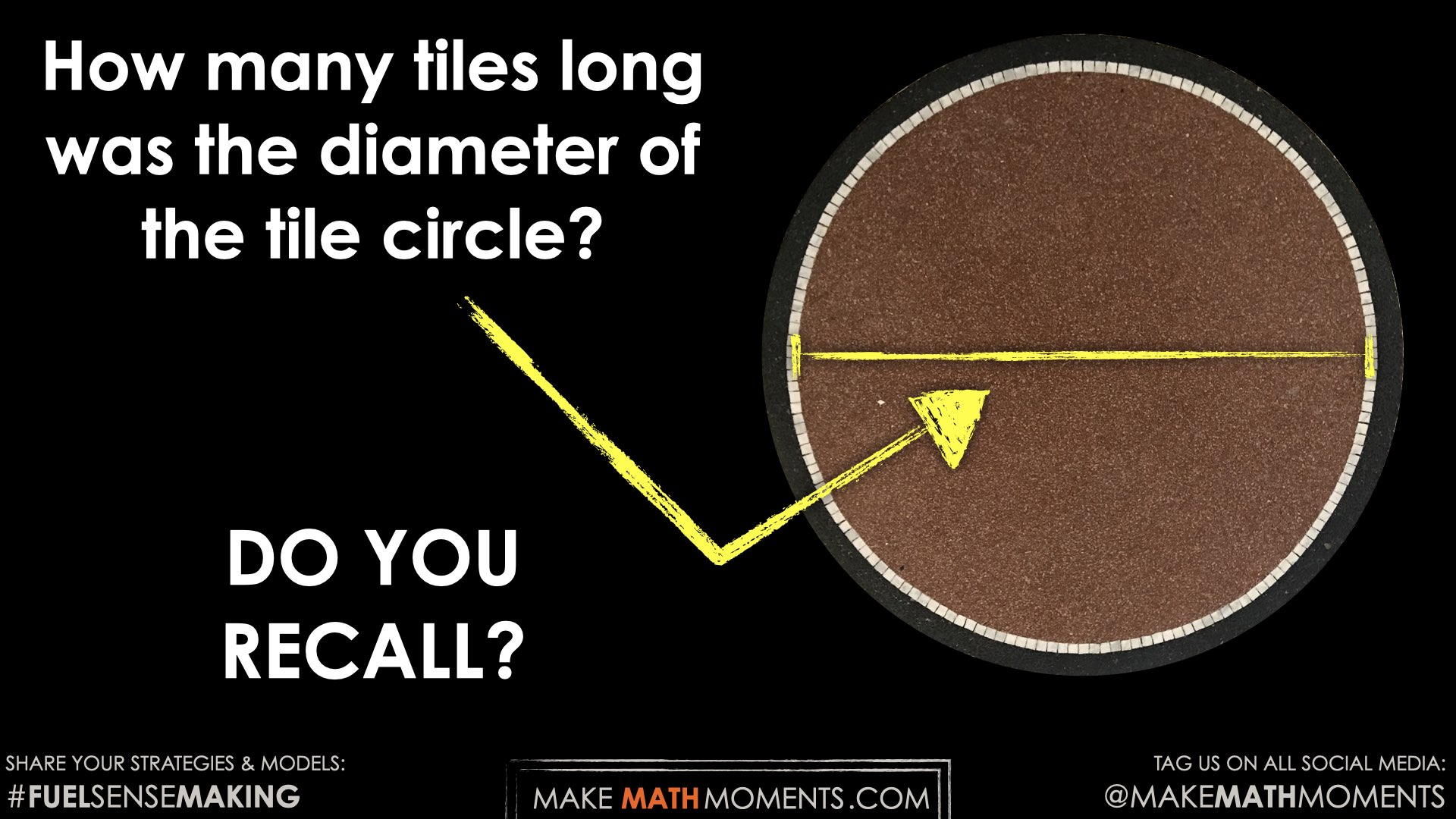Once students have shared that it was 52 square tiles, you can ask them to either recall or approximate how many tiles it would take to wrap around the circumference of the circle (164 square tiles).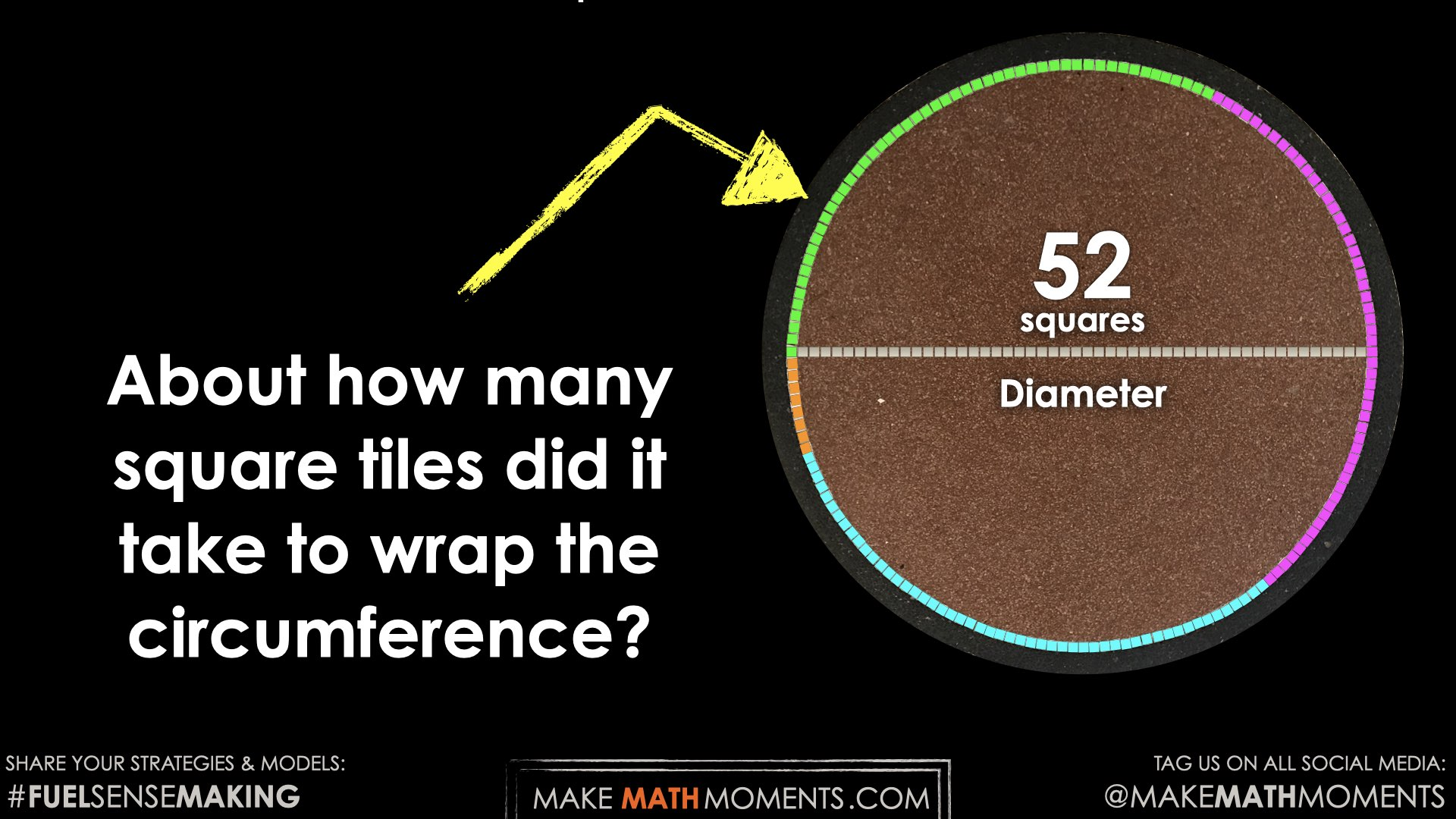Ask them to share approximately how many times larger the circumference was than the diameter, known as Pi before confirming that the length of the circumference is approximately 164 square tiles.

Then, share the following prompt with students to spark curiosity and promote wonderment:

How many square tiles would it take to fill the Tile Circle?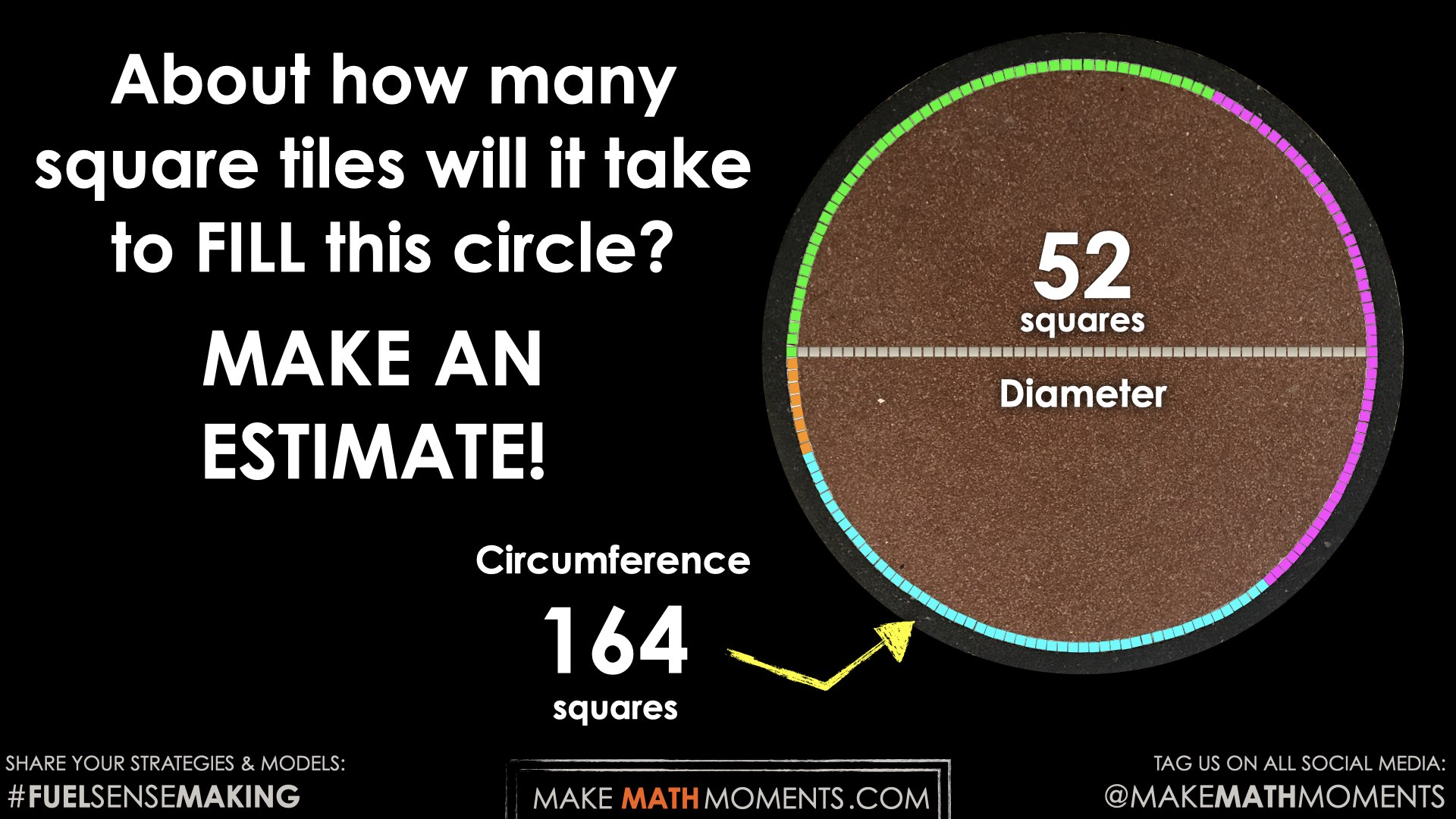Ask students to use only mental math without yet drawing models. This is a good opportunity for students to reflect on what was done in the previous lesson and to see if they can do any decomposing of the circle in their mind.

You can also prompt them with the following:

Think of 3 numbers:

• a number you think is too low;
• a number you think is too high; and,
• a number you think is just right.

Give students time to turn and talk to share with each other, then share out to the math community.

## Crafting A Productive Struggle: Prompt

After students have shared their low, high and just right estimates and you have recorded them on the board, chart paper or a digital collaboration space, you can then prompt them to attempt becoming more precise:

About how many square tiles will it take to fill the Tile Circle?
Convince us by modelling your thinking.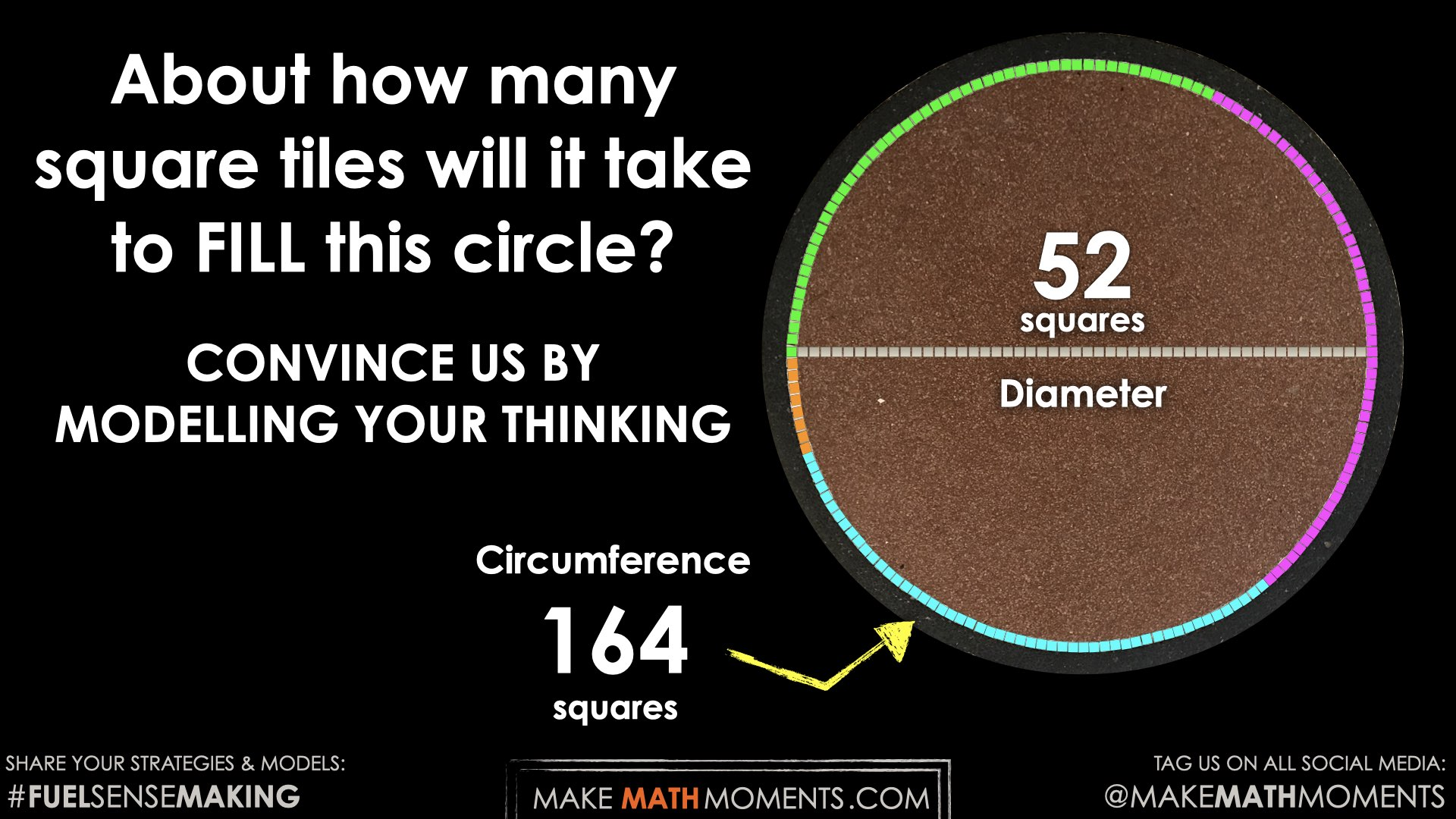We are still going to encourage students to avoid the use of a calculator and if a student is relying on a formula alone, we will prompt them to help convince us because we aren’t sure if we can trust the formula yet.

## While Students Are Productively Struggling…

Login/Join to access the entire Teacher Guide, downloadable slide decks and printable handouts for this lesson and all problem based units.

## Student Approach #1: Decomposing Circle Cutout and Recomposing Over Grid Paper

Login/Join to access the entire Teacher Guide, downloadable slide decks and printable handouts for this lesson and all problem based units.

## Student Approach #2: Decomposing Circle Cutout and Recomposing Over Grid Paper

Login/Join to access the entire Teacher Guide, downloadable slide decks and printable handouts for this lesson and all problem based units.

## Student Approach #3: Using a Formula for Area of a Circle

Login/Join to access the entire Teacher Guide, downloadable slide decks and printable handouts for this lesson and all problem based units.

## Reveal

Now that students have shared their thinking and have convinced others of about how many square tiles it would take to fill the Tile Circle, you can show the following silent solution reveal and consolidation video:

Here are a few screenshots from the final frames of the reveal video: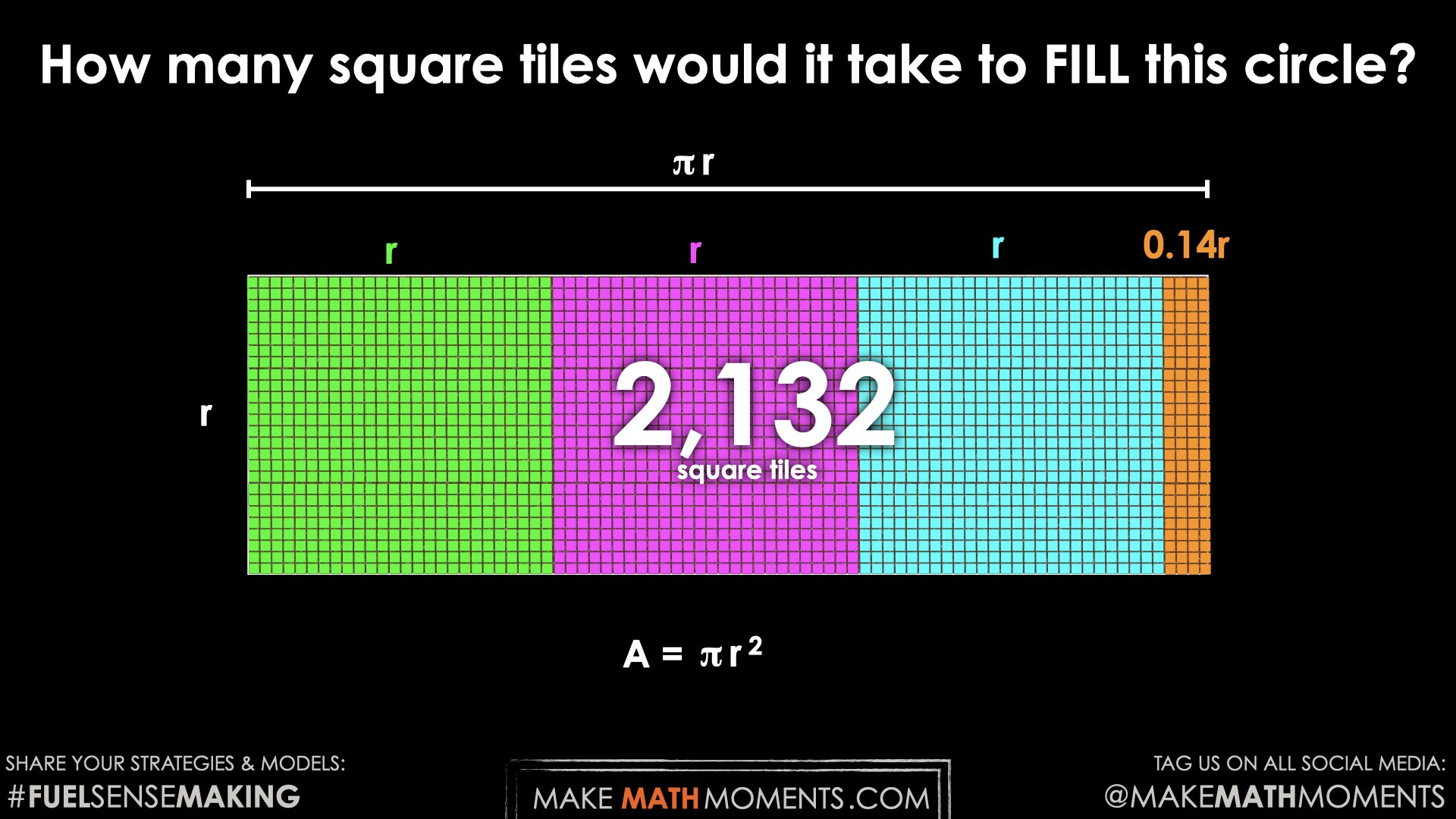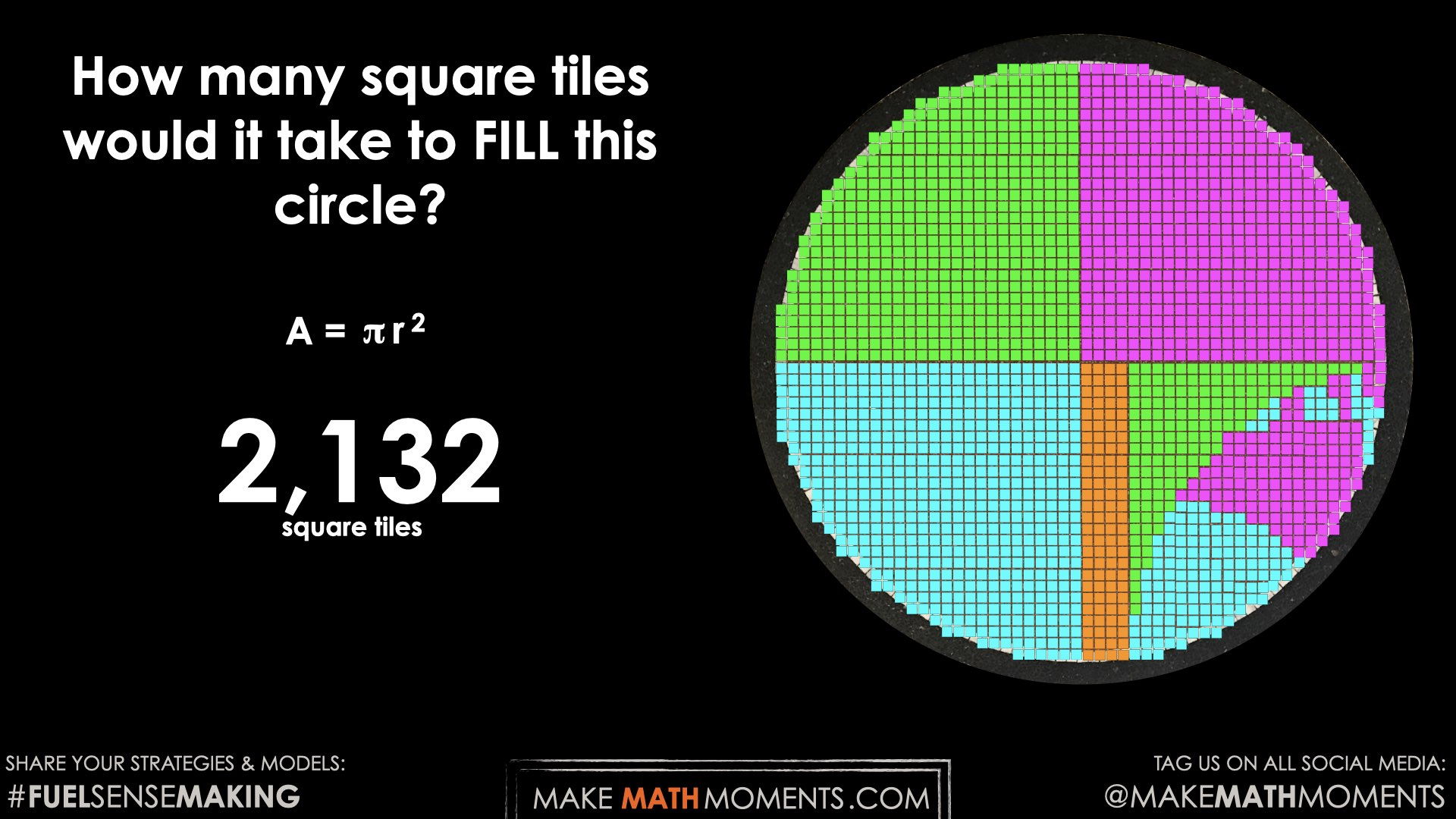Facilitator Note:

Our final answer is based on an approximate circumference of 164 units whereas students who use a more precise circumference of say πd ≈ (3.14)(52 units) ≈ 163.28 will result in an area closer to 2122.64 square units regardless of whether they use the standard area of a circle formula, A = $$πr^2$$, or our decomposed circle/recomposed rectangle approach, A = (½ of the circumference)(radius).

The reasoning for rounding the circumference up to a whole number of 164 units vs. down to 163 units was to avoid the need for a half unit throughout this investigation.

## Consolidation

Login/Join to access the entire Teacher Guide, downloadable slide decks and printable handouts for this lesson and all problem based units.

## Reflect and Consolidation Prompts

Provide students an opportunity to reflect on their learning by offering these consolidation prompts to be completed independently.

### Consolidation Prompt #1:

Login/Join to access the entire Teacher Guide, downloadable slide decks and printable handouts for this lesson and all problem based units.

### Consolidation Prompt #2:

Login/Join to access the entire Teacher Guide, downloadable slide decks and printable handouts for this lesson and all problem based units.

We suggest collecting this reflection as an additional opportunity to engage in the formative assessment process to inform next steps for individual students as well as how the whole class will proceed.

Login/Join to access the entire Teacher Guide, downloadable slide decks and printable handouts for this lesson and all problem based units.

## Educator Discussion Area

Login/Join to access the entire Teacher Guide, downloadable slide decks and printable handouts for this lesson and all problem based units.

## Explore Our 60+ Problem Based Units

This Make Math Moments Lesson was designed to spark curiosity for a multi-day unit of study with built in purposeful practice, number talks and extensions to elicit and emerge strategies and mathematical models.

Dig into our other units of study and view by concept continuum, grade or topic!